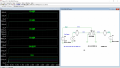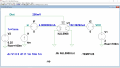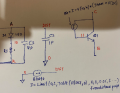# SPICE MODEL PS2561F

#### sunney

Joined Sep 3, 2015
14
Hi,

I was trying to model a reflective type optocoupler from NJR p/n NJL5908AR.
Unfortunately NJR doesn't have spice model available for these parts.

While I was searching for spice model on web, I found an application note by CEL (could not attach for some reason) for PS2561F optocoupler, thinking that I will be able to model reflective type optocoupler by taking reference from PS2561F model.

Note: Application note is from California Electronics Laboratories, AN3017
The spice model code is pasted at the end.

The statement which I don't understand is:
"Gctr C B TABLE
+ {If(V(T)<3m,
+ ((-94570000000*PWR(V(T),5)+694000000*PWR(V(T),4)
+ -1951000*PWR(V(T),3)+2918*PWR(V(T),2)-0.2827*V(T)+0.00001958)*0.92/550),
+ ((-24590*PWR(V(T),4)+3484*PWR(V(T),3)-178.1*PWR(V(T),2)+3.983*V(T)
+ -0.004788)*0.96/550))}
+ (0,0) (0.1m, 0.1m)"

This is (I suppose) the polynomial equation for CTR (Current transfer ratio) and multiplying CTR values with the forward current (in terms of voltage) using behavioural model.

Now since the part from NJR NJL5908AR doesn't have CTR mentioned since its a reflective type I guess, I have drawn a graph by using the characteristics graph b/w output current vs Forward current and derive the polynomial using trend line property of excel, but I am not getting proper simulation results.

SPICE MODEL Below:
* the maximum ratings as called out in the data sheet.
*
* Please note that the model can't duplicate exact device performance in the
* real world under all conditions and they can't replace the breadboarding
* for final design verification.
*
* Note: CTR is modelled base on sample A in the data sheet. Please refer to the
* data sheet for details.
*********************************************************************************
* A = PIN 1: diode anode
* K = PIN 2: diode cathode
* E = PIN 3: BJT emitter
* C = PIN 4: BJT collector
.SUBCKT PS2381 A K E C
D1 A D LED
Vsense D K 0
Hd R 0 Vsense 1
Rd R T 100k
Cd T 0 20p
Gctr C B TABLE
+ {If(V(T)<3m,
+ ((-94570000000*PWR(V(T),5)+694000000*PWR(V(T),4)
+ -1951000*PWR(V(T),3)+2918*PWR(V(T),2)-0.2827*V(T)+0.00001958)*0.92/550),
+ ((-24590*PWR(V(T),4)+3484*PWR(V(T),3)-178.1*PWR(V(T),2)+3.983*V(T)
+ -0.004788)*0.96/550))}
+ (0,0) (0.1m, 0.1m)
Q1 C B E detector
.model LED D IS=1p N=1.999644 RS=0 BV=6 IBV=10u
+ CJO=10p EG=1.424 TT=500n
.model detector NPN IS=100p BF=550 NF=1.3 BR=50 TF=2n TR=1.5n
+ CJE=15p CJC=20p VAF=100 ISS=0 CJS=1p
.ends
*\$

sunney

#### eetech00

Joined Jun 8, 2013
2,197
Hello

I've taken an existing LTspice opto and modified it for the current transfer characteristics of the NJL5908. I sometimes find its easier to modify an existing model than to create a new one. I've added a "distance" pin where a voltage can be applied to vary the distance from the reflected surface to the part. Distance scale is 1V=1mm.

The sim shows the DC opt of the part with "Dist" set to equal 100%. Comment out the .op statement, and Uncomment the .dc statement to view the Output characteristics plot.

Output Characteristics Plot:DC op plot:•Bordodynov, sunney and ericgibbs

#### sunney

Joined Sep 3, 2015
14
Hi eetech00,

I appreciate all your support and thanks a lot for modelling optocoupler. I would like to have the files which you have created.

Thank you,
Sunney

#### eetech00

Joined Jun 8, 2013
2,197
Hi eetech00,

I appreciate all your support and thanks a lot for modelling optocoupler. I would like to have the files which you have created.

Thank you,
Sunney
Here you go.
Model, symbol and test files attached.

Note to moderator:
You might want to rename this thread:
"SPICE MODEL for NJL5908"

#### Attachments

• 2.1 KB Views: 3
•Bordodynov and sunney

#### sunney

Joined Sep 3, 2015
14
Here you go.
Model, symbol and test files attached.

Note to moderator:
You might want to rename this thread:
"SPICE MODEL for NJL5908"
Hi eetech00,

I have downloaded the files and it is working as you have already shown. Again thankyou for that.

Since I was earlier going through some of other spice model for optocouplers, I had started drawing some sort of schematic based on the net list given in the code. Same thing I did with your spice model to understand more about writing spice programs.

I have few questions related to that :

1. Please check whether the schematic I have drawn is correct or not.
2. From where the "Igain = 0.065m" value came. Params: Igain = 0.065m, what does Params means here.
The reason I asked this because gain (or CTR) which I tried to simulate earlier was from graph between Iout and IF i.e. Iout/IF, but it wasn't linear so I had to use some sort of TABLE function in behavioral model as you have used for simulating the distance function.
3. While defining BJT, why there is an extra letter 'E' or I am missing something i.e. Q1 C 11 E E NP
4. .model statement used for LED, whether the parameters were deduced from the datasheet or you have used standard .model for diode. as BV = 6V which seems to be taken from datasheet, but not sure how Is (saturation current), N (emission coefficient [1to 2] etc are selected.
5. .model statement used for BJT, again how the parameters are derived.I know I am asking lot of questions, but not sure how to find answers for these other than asking you.

Thanks,
Sunney

#### sunney

Joined Sep 3, 2015
14
Hi eetech00,

Awaiting your response, it will help me to understand the model.

Regards,
Sunney

#### eetech00

Joined Jun 8, 2013
2,197
Hi eetech00,

I have downloaded the files and it is working as you have already shown. Again thankyou for that.

Since I was earlier going through some of other spice model for optocouplers, I had started drawing some sort of schematic based on the net list given in the code. Same thing I did with your spice model to understand more about writing spice programs.

I have few questions related to that :

1. Please check whether the schematic I have drawn is correct or not.
Looks correct, except there should be a "hidden" 1 ohm resistor and 1p Cap in parallel with BDist.

2. From where the "Igain = 0.065m" value came. Params: Igain = 0.065m, what does Params means here.
The reason I asked this because gain (or CTR) which I tried to simulate earlier was from graph between Iout and IF i.e. Iout/IF, but it wasn't linear so I had to use some sort of TABLE function in behavioral model as you have used for simulating the distance function.
The "params: Igain" option creates the Igain parameter and makes it externally available to the user as well as the scope of the subcircuit definition.
If you don't want the user to be able to set it (and you probably dont), remove the "params: Igain" parameter and then hard code the value into the BG statement. Again, I just left it there because it was already in the template.

I used a existing PC817 model as a template, replaced the original diode with one I modeled, and added the distance function using the datasheet. I then, after checking the transistor was suitable (using the datasheet), I tuned the gain until I obtained the correct CTR.

3. While defining BJT, why there is an extra letter 'E' or I am missing something i.e. Q1 C 11 E E NP
The second E represents the transistor substrate, and for this application, the emitter and substrate are connected together.
If you use the three terminal NPN transistor symbol, LTspice will automatically connect the substrate to 0 (zero or ground) by default. I left it there because it was already there.

4. .model statement used for LED, whether the parameters were deduced from the datasheet or you have used standard .model for diode. as BV = 6V which seems to be taken from datasheet, but not sure how Is (saturation current), N (emission coefficient [1to 2] etc are selected.
There are plenty of documents and methods for modeling diodes. Search the internet for "LED or Diode Modeling".

5. .model statement used for BJT, again how the parameters are derived.

Last edited:
•sunney

#### sunney

Joined Sep 3, 2015
14
Looks correct, except there should be a "hidden" 1 ohm resistor and 1p Cap in parallel with BDist.

The "params: Igain" option creates the Igain parameter and makes it externally available to the user as well as the scope of the subcircuit definition.
If you don't want the user to be able to set it (and you probably dont), remove the "params: Igain" parameter and then hard code the value into the BG statement. Again, I just left it there because it was already in the template.

I used a existing PC817 model as a template, replaced the original diode with one I modeled, and added the distance function using the datasheet. I then, after checking the transistor was suitable (using the datasheet), I tuned the gain until I obtained the correct CTR.

The second E represents the transistor substrate, and for this application, the emitter and substrate are connected together.
If you use the three terminal NPN transistor symbol, LTspice will automatically connect the substrate to 0 (zero or ground) by default. I left it there because it was already there.

There are plenty of documents and methods for modeling diodes. Search the internet for "LED or Diode Modeling".